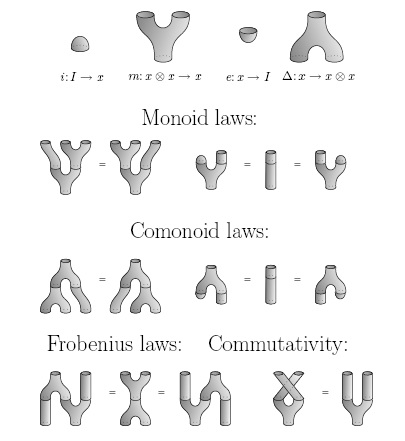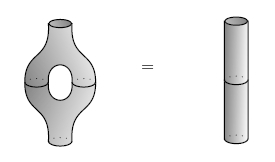## June 19, 2013

### A Characterization of Relative Entropy (Part 1)

#### Posted by John BaezI’m trying to finish off a paper that Tobias Fritz and I have been working on, which gives a category-theoretic (and Bayesian!) characterization of relative entropy. It’s a kind of sequel to our paper with Tom Leinster, in which we characterized entropy.

That earlier paper was developed in conversations here on the n-Category Café. It was a lot of fun; I sort of miss that style of working. Also, to get warmed up, I need to think through some things I’ve thought about before. So, I might as well write them down here.

There are many categories related to probability theory, and they’re related in many ways. Last summer—on the 24th of August 2012, according to my notes here—Jamie Vicary, Brendan Fong and I worked through a bunch of these relationships. I need to write them down now, even if they’re not all vitally important to my paper with Tobias. They’re sort of buzzing around my brain like flies.

(Tobias knows this stuff too, and this is how we think about probability theory, but we weren’t planning to stick it in our paper. Maybe we should.)

Let’s restrict attention to probability measures on finite sets, and related structures. We could study these questions more generally, and we should, but not today. What we’ll do is give a unified purely algebraic description of:

• finite sets
• measures on finite sets
• probability measures on finite sets
• functions
• bijections
• measure-preserving functions
• stochastic maps

### Finitely generated free $\left[0,\infty \right)$-modules

We start with the rig of nonnegative real numbers with their usual addition and multiplication; let’s call this $\left[0,\infty \right)$. The idea is that measure theory, and probability theory, are closely related to linear algebra over this rig.

So, we start with the category of finitely generated free $\left[0,\infty \right)$-modules, and module homomorphisms. Every such module is isomorphic to $\left[0,\infty {\right)}^{S}$ for some finite set $S$.

Puzzle. Do we need to say ‘free’ here? Are there finitely generated modules over $\left[0,\infty \right)$ that aren’t free?

In other words, every finitely generated free $\left[0,\infty \right)$-module is isomorphic to $\left[0,\infty {\right)}^{n}$ for some $n=0,1,2,\dots$. So, the category of these is equivalent to $\mathrm{Mat}\left(\left[0,\infty \right)\right)$, the category where objects are natural numbers, a morphism from $m$ to $n$ is an $m×n$ matrix of numbers in $\left[0,\infty \right)$, and composition is done by matrix multiplication. So let’s just call this category $\mathrm{Mat}\left(\left[0,\infty \right)\right)$, instead of something complicated like ${\mathrm{FinGenFMod}}_{\left[0,\infty \right)}$. I’ll call the morphisms in here maps.

We can take tensor products of finitely generated free modules, and this makes $\mathrm{Mat}\left(\left[0,\infty \right)\right)$ into a symmetric monoidal $†$-category. This means we can draw maps using string diagrams in the usual way. However, I’m feeling lazy so I’ll often write equations when I could be drawing diagrams.

One of the rules of the game is that all these equations will make sense in any symmetric monoidal $†$-category. So we could, if we wanted, generalize ideas from probability theory this way. If you want to do this, you’ll need to know that $\left[0,\infty \right)$ is the unit for the tensor product in the category of finitely generated free $\left[0,\infty \right)$-algebras. We’ll be seeing this guy a lot. So if you want to generalize, just call it ‘the tensor unit’.

### Finite sets

There’s a way to see the category of finite sets lurking in $\mathrm{Mat}\left(\left[0,\infty \right)\right)$, which we can borrow from this paper:

For any finite set $S$, we get a free finitely generated $\left[0,\infty \right)$-module, namely $\left[0,\infty {\right)}^{S}$. This comes with some structure:

• a multiplication $m:\left[0,\infty {\right)}^{S}\otimes \left[0,\infty {\right)}^{S}\to \left[0,\infty {\right)}^{S}$, coming from pointwise multiplication of $\left[0,\infty \right)$-valued functions on $S$;
• the unit for this multiplication, an element of $\left[0,\infty {\right)}^{S}$, which we can write as a morphism $i:\left[0,\infty \right)\to \left[0,\infty {\right)}^{S}$;
• a comultiplication, obtained by taking the diagonal map $\Delta :S\to S×S$ and promoting it to a linear map $\Delta :\left[0,\infty {\right)}^{S}\to \left[0,\infty {\right)}^{S}\otimes \left[0,\infty {\right)}^{S}$;
• a counit for this comultiplication, obtained by taking the map to the terminal set $!:S\to 1$ and promoting it to a linear map $e:\left[0,\infty {\right)}^{S}\to \left[0,\infty \right)$.

These morphisms $m,i,\Delta ,e$ make

$x=\left[0,\infty {\right)}^{S}$

into a commutative Frobenius algebra in $\mathrm{Mat}\left(\left[0,\infty \right)\right)$. That’s a thing where the unit, counit, multiplication and comultiplication obey these laws:(I drew these back when I was feeling less lazy.) This Frobenius algebra is also ‘special’, meaning it obeys this:And it’s also a $†$-Frobenius algebra, meaning that the counit and comultiplication are obtained from the unit and multiplication by ‘flipping’ them using the $†$-category structure. (If we think of a morphism in $\mathrm{Mat}\left(\left[0,\infty \right)\right)$ as a matrix, its dagger is its transpose.)

Conversely, suppose we have any special commutative $†$-Frobenius algebra $x$. Then using the ideas in the paper by Coecke, Pavlovich and Vicary we can recover a basis for $x$, consisting of the vectors ${e}_{i}\in x$ with

$\Delta \left({e}_{i}\right)={e}_{i}\otimes {e}_{i}$

This basis forms a set $S$ such that

$x\cong \left[0,\infty {\right)}^{S}$

for some specified isomorphism in $\mathrm{Mat}\left(\left[0,\infty \right)\right)$. Furthermore, this is an isomorphism of special commutative $†$-Frobenius algebras!

In short, a special commutative $†$-Frobenius algebra in $\mathrm{Mat}\left(\left[0,\infty \right)\right)$ is just a fancy way of talking about a finite set.

### Functions and bijections

Now suppose we have two special commutative $†$-Frobenius algebra in $\mathrm{Mat}\left(\left[0,\infty \right)\right)$: $x$ and $y$.

Suppose $f:x\to y$ is a Frobenius algebra homomorphism: that is, a map preserving all the structure—the unit, counit, multiplication and comultiplication. Then it comes from an isomorphism of finite sets. This lets us find ${\mathrm{FinSet}}_{0}$, the groupoid of finite sets and bijections, inside $\mathrm{Mat}\left(\left[0,\infty \right)\right)$.

Alternatively, suppose $f:x\to y$ is just a coalgebra homomorphism: that is a map preserving just the counit and comultiplication. Then it comes from an arbitrary function between finite sets. This lets us find $\mathrm{FinSet}$, the category of finite sets and functions, inside $\mathrm{Mat}\left(\left[0,\infty \right)\right)$.

If $f$ preserves the comultiplication, it automatically preserves the counit. But what if it preserves just the counit? This sounds like a dry, formal question. But it’s not: the answer is something useful, a ‘stochastic map’.

### Stochastic maps

A stochastic map from a finite set $S$ to a finite set $T$ is a map sending each point of $S$ to a probability measure on $T$.

We can think of this as a $T×S$-shaped matrix of numbers in $\left[0,\infty \right)$, where a given column gives the probability that a given point in $S$ goes to any point in $T$. The sum of the numbers in each column will be 1. And conversely, any $T×S$-shaped matrix of numbers in $\left[0,\infty \right)$, where each column sums to 1, gives a stochastic map from $S$ to $T$.

But now let’s describe this idea using the category $\mathrm{Mat}\left(\left[0,\infty \right)\right)$. We’ve seen a finite set is the same as a special commutative $†$-Frobenius algebra. So, say we have two of these, $x$ and $y$. Our matrix of numbers in $\left[0,\infty \right)$ is just a map

$f:x\to y$

So, we just need a way to state the condition that each column in the matrix sums to 1. And this condition simply says that $f$ preserves the counit:

${ϵ}_{y}\circ f={ϵ}_{x}$

where ${ϵ}_{x}:x\to \left[0,\infty \right)$ is the counit for $x$, and similarly for ${ϵ}_{y}$.

To understand this, note that if we use the canonical isomorphism

$x\cong \left[0,\infty {\right)}^{S}$

the counit ${ϵ}_{x}$ can be seen as the map

$\left[0,\infty {\right)}^{S}\to \left[0,\infty \right)$

that takes any $S$-tuple of numbers and sums them up. In other words, it’s integration with respect to counting measure. So, the equation

${ϵ}_{y}\circ f={ϵ}_{x}$

says that if we take any $S$-tuple of numbers, multiply it by the matrix $f$, and then sum up the entries of the resulting $T$-tuple, it’s the same as if we summed up the original $S$-tuple. But this says precisely that each column of the matrix $f$ sums to 1.

### Finite measure spaces

Now let’s use our formalism to describe finite measure spaces—by which, beware, I mean a finite sets equipped with measures! To do this, we’ll use a special commutative $†$-Frobenius algebra $x$ in $\mathrm{Mat}\left(\left[0,\infty \right)\right)$ together with any map

$\mu :\left[0,\infty \right)\to x$

Starting from these, we get a specified isomorphism

$x\cong \left[0,\infty {\right)}^{S}$

and $\mu$ sends the number 1 to a vector in $\left[0,\infty {\right)}^{S}$: that is, a function on $S$ taking values in $\left[0,\infty \right)$. Multiplying this function by counting measure, we get a measure on $S$.

Puzzle. How can we describe this measure without the annoying use of counting measure?

Conversely, any measure on a finite set gives a special commutative $†$-Frobenius algebra $x$ in $\mathrm{Mat}\left(\left[0,\infty \right)\right)$ equipped with a map from $\left[0,\infty \right)$.

So, we can say a finite measure space is a special commutative $†$-Frobenius algebra in $\mathrm{Mat}\left(\left[0,\infty \right)\right)$ equipped with a map

$\mu :\left[0,\infty \right)\to x$

And given two of these,

$\mu :\left[0,\infty \right)\to x,\phantom{\rule{2em}{0ex}}\nu :\left[0,\infty \right)\to y$

and a coalgebra morphism

$f:x\to y$

such that the obvious triangle commutes:

$f\circ \mu =\nu$

then we get a measure-preserving function between finite measure spaces! Conversely, any measure-preserving function between finite measure spaces gives us such a commutative triangle.

So, we get a way of describing the category $\mathrm{FinMeas}$, with finite measure spaces as objects and measure-preserving maps as objects.

### Finite probability measure spaces

I’m mainly interested in probability measures. So suppose $x$ is a special commutative $†$-Frobenius algebra in $\mathrm{Mat}\left(\left[0,\infty \right)\right)$ equipped with a map

$\mu :\left[0,\infty \right)\to x$

We’ve seen this gives a finite measure space. But this is a probability measure space if and only if

$e\circ \mu =1$

where

$e:x\to \left[0,\infty \right)$

is the counit for $x$. The equation simply says the total integral of our measure $\mu$ is 1.

So, we get a way of describing the category $\mathrm{FinProb}$, with finite probability measure spaces as objects and measure-preserving maps as objects. Given finite probability measure spaces described this way:

$\mu :\left[0,\infty \right)\to x,\phantom{\rule{2em}{0ex}}\nu :\left[0,\infty \right)\to y$

a measure-preserving function is a coalgebra morphism

$f:x\to y$

such that the obvious triangle commutes:

$f\circ \mu =\nu$

### Measure-preserving stochastic maps

Say we have two finite measure spaces. Then we can ask whether a stochastic map from one to the other is measure-preserving. And we can answer this question in the language of $\mathrm{Mat}\left(\left[0,\infty \right)\right)$.

Remember, a finite measure space is a special commutative $†$-Frobenius algebra $x$ in $\mathrm{Mat}\left(\left[0,\infty \right)\right)$ together with a map

$\mu :\left[0,\infty \right)\to x$

Say we have another one:

$\nu :\left[0,\infty \right)\to y$

A stochastic map is just a map

$f:x\to y$

that preserves the counit:

${ϵ}_{y}\circ f={ϵ}_{x}$

But it’s a measure-preserving stochastic map if also

$f\circ \mu =\nu$

### Next…

There’s a lot more to say; I haven’t gotten anywhere near what Tobias and I are doing! But it’s pleasant to have this basic stuff written down.

Posted at June 19, 2013 11:02 PM UTC

TrackBack URL for this Entry:   http://golem.ph.utexas.edu/cgi-bin/MT-3.0/dxy-tb.fcgi/2627